## 傅里叶变换

### 傅里叶变换的定义

`$F\left({\omega }_{1},{\omega }_{2}\right)=\sum _{m=-\infty }^{\infty }\sum _{n=-\infty }^{\infty }f\left(m,n\right){e}^{-j{\omega }_{1}m}{e}^{-j{\omega }_{2}n}.$`

`$f\left(m,n\right)=\frac{1}{4{\pi }^{2}}{\int }_{{\omega }_{1}=-\pi }^{\pi }{\int }_{{\omega }_{2}=-\pi }^{\pi }F\left({\omega }_{1},{\omega }_{2}\right){e}^{j{\omega }_{1}m}{e}^{j{\omega }_{2}n}d{\omega }_{1}d{\omega }_{2}.$`

#### 傅里叶变换的可视化`$|F\left({\omega }_{1},{\omega }_{2}\right)|,$`

。幅值的网格图是可视化傅里叶变换的常用方法。`$\mathrm{log}|F\left({\omega }_{1},{\omega }_{2}\right)|$`F(ω12) 非常接近 0 的区域，使用对数有助于显示傅里叶变换的细节。### 离散傅里叶变换

• DFT 的输入和输出均为离散值，便于计算机操作。

• 有一种计算 DFT 的快速算法，称为快速傅里叶变换 (FFT)。

DFT 通常针对仅在有限区域 $0\le m\le M-1$$0\le n\le N-1$ 上为非零值的离散函数 f(m,n) 定义。二维 M×N DFT 和逆 M×N DFT 的关系由下式给出：

F(p,q)f(m,n) 的 DFT 系数。零频率系数 F(0,0) 通常称为“DC 分量”。DC 是一个电气工程术语，表示直流电。（请注意，MATLAB® 中的矩阵索引始终从 1 开始，而不是从 0 开始；因此，矩阵元素 f(1,1)F(1,1) 分别对应于数学量 f(0,0)F(0,0)。）

MATLAB 函数 `fft``fft2``fftn` 分别实现用于计算一维 DFT、二维 DFT 和 N 维 DFT 的快速傅里叶变换算法。函数 `ifft``ifft2``ifftn` 计算逆 DFT。

#### 与傅里叶变换的关系

DFT 系数 F(p,q) 是傅里叶变换 F(ω12) 的采样。

`$\begin{array}{cc}F\left(p,q\right)=F\left({\omega }_{1},{\omega }_{2}\right){|}_{\begin{array}{l}{\omega }_{1}=2\pi p/M\\ {\omega }_{2}=2\pi q/N\end{array}}& \begin{array}{l}p=0,1,...,M-1\\ q=0,1,...,N-1\end{array}\end{array}$`

#### 离散傅里叶变换的可视化

1. 构造矩阵 `f`，它类似于傅里叶变换的定义的示例中的函数 f(m,n)。前面提到过，f(m,n) 在矩形区域内等于 1，在其他位置等于 0。使用二值图像来表示 f(m,n)

```f = zeros(30,30); f(5:24,13:17) = 1; imshow(f,'InitialMagnification','fit')```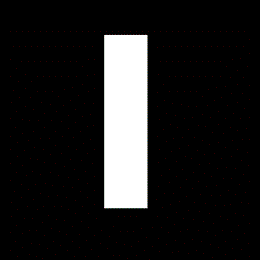2. 使用以下命令计算并可视化 `f` 的 30×30 DFT。

```F = fft2(f); F2 = log(abs(F)); imshow(F2,[-1 5],'InitialMagnification','fit'); colormap(jet); colorbar```

在无填充情况下计算的离散傅里叶变换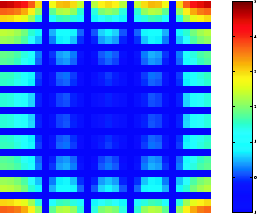该图不同于傅里叶变换的可视化中显示的傅里叶变换。首先，傅里叶变换的采样要粗略得多。其次，零频系数显示在左上角，而不是传统的中心位置。

3. 为了获得傅里叶变换的更精细采样，在计算 `f` 的 DFT 时可向在其中填零。使用以下命令，可一步执行填零和 DFT 计算。

`F = fft2(f,256,256);`

该命令在计算 DFT 之前用零将 `f` 填充为 256×256。

`imshow(log(abs(F)),[-1 5]); colormap(jet); colorbar`

在填充情况下计算的离散傅里叶变换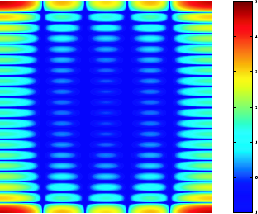4. 然而，零频系数仍显示在左上角，而不是中心位置。您可以使用函数 `fftshift` 解决此问题，该函数交换 `F` 的象限，使零频系数位于中心位置。

```F = fft2(f,256,256);F2 = fftshift(F); imshow(log(abs(F2)),[-1 5]); colormap(jet); colorbar```

生成的图与傅里叶变换的可视化中所示的图相同。

### 傅里叶变换的应用

#### 线性滤波器的频率响应

```h = fspecial('gaussian'); freqz2(h)```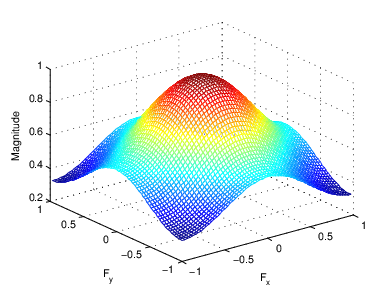#### 使用傅里叶变换执行快速卷积

```A = magic(3); B = ones(3);```

`A``B` 执行填零，使它们至少为 (M+P-1)×(N+Q-1)。（通常填零后的 `A``B` 的大小要为 2 的幂，这时 `fft2` 的执行速度最快。）该示例将矩阵填充为 8×8。

```A(8,8) = 0; B(8,8) = 0;```

`C = ifft2(fft2(A).*fft2(B));`

```C = C(1:5,1:5); C = real(C)```
```C = 5×5 8.0000 9.0000 15.0000 7.0000 6.0000 11.0000 17.0000 30.0000 19.0000 13.0000 15.0000 30.0000 45.0000 30.0000 15.0000 7.0000 21.0000 30.0000 23.0000 9.0000 4.0000 13.0000 15.0000 11.0000 2.0000 ```

#### 执行基于 FFT 的相关性分析来定位图像特征

`bw = imread('text.png');`

`a = bw(32:45,88:98);`

```C = real(ifft2(fft2(bw) .* fft2(rot90(a,2),256,256))); figure imshow(C,[]) % Scale image to appropriate display range.```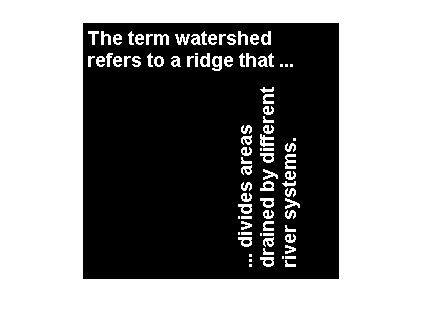`max(C(:))`
```ans = 68 ```
```thresh = 60; % Use a threshold that's a little less than max. D = C > thresh; se = strel('disk',5); E = imdilate(D,se); figure imshow(E) % Display pixels with values over the threshold.```